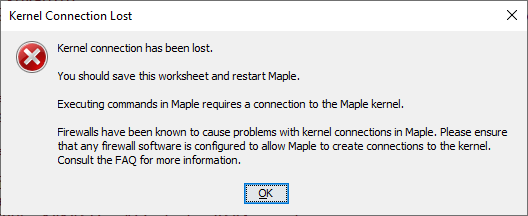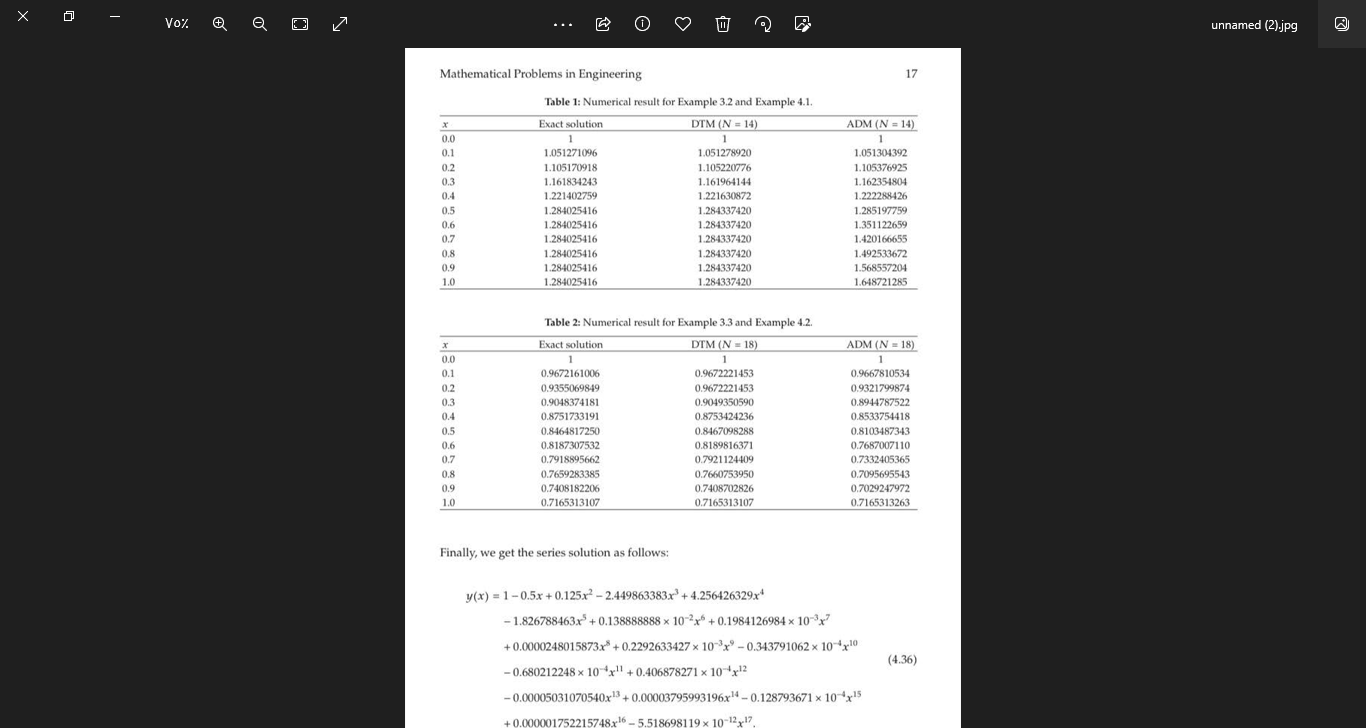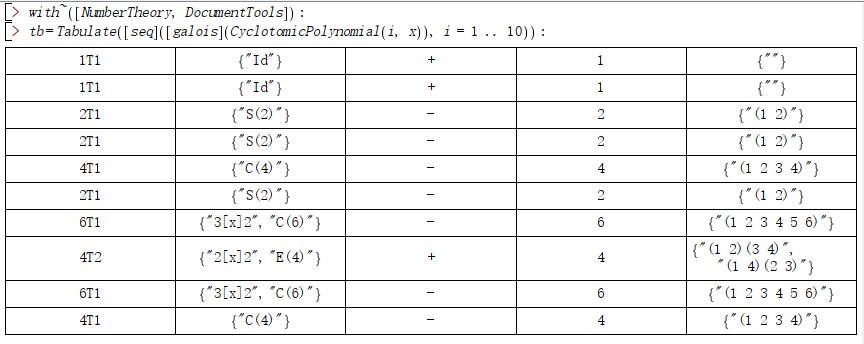## nyqlog for Maple...

Anyone out there converted the  nyqlog at MATLAB/nyqlog.m at master · nielsSkov/MATLAB · GitHub  to Maple or Maple Flow? For Nyquist plots...

## Réalisation complète ...

Bonjour, petite question simple que je me pose la MapleSim permet de tout réaliser ?

## Maple noob need help !!!!!...

Hello everyone !

I am completely new to using math solving applications like maple.

I was introduced to it in my university course and since then I found it very helpful in solving 2nd degree differential equations and integrals.

However, i don't have any intuition for programming, so i found the commands of maple very confusing.

The last couple of weeks, I have been completely destroyed by a problem I've recently encountered...

I was given a bunch of (x,y) coordinates of a function and i was told that the function in question is a polynomial of a 3rd degree.

So my question is, can maple help me in solving this type of problems or no ?!?!?!

And if yes, can someone give me a general direction in which i have to go ?

Also, I was told that this problem can be solved in Excel ?

Thank you all in adavce !

## Non Linear System solved using solve and solve...

Looking for the solution of this a non linear system y = x^2 + 2*x - 3 ,  y = x^3 + 3*x^2 - 4*x - 8 (The system has 3 real solutions A = (-3.35, 1.51)    B = (-0.72, -3.92)  C = (2.07, 5.42) ).

i have tried to solve it as follows:

eq1 := x^2 + 2*x - 3

eq2 := x^3 + 3*x^2 - 4*x - 8

fsolve({eq1 = eq2})

I get

{x = -3.346462190}, {x = -0.7222456338}, {x = 2.068707823}

That is what i expected.

But if i try to use solve and then evalf i find three complex solutions.

solution := solve({eq1 = eq2}, explicit)

evalf(%);

{ x = 2.068707824 - 4x10^(-10)   I },     { x = -3.346462190 - 7.660254040 x10^(-10)    I },   { x = -0.722245634 + 9.660254040x10^(-10)    I }

Why ? I don't understand.

Moreover, solving the equations as follows:
sys1 := y = x^2 + 2*x - 3;
sys2 := y = x^3 + 3*x^2 - 4*x - 8;

fsolve({sys1, sys2}, {x, y})

yeld just one solution:

{x = 2.068707823, y = 5.416967705}

Someone can explain me this behaviour ?

## How to extract dat file from contour plot...

Dear Users,

I hope everyone here is fine. I want to extract dat file from the attached contour plot file. Please help me to resolve this issue. Many thanks in advance

Dat_file.mw

## why so many "kernel connection has been lost" in M...

I get about 3-4 of these each day when I run my long script. Maple 2022.2 on windows 10They happen much more for me in Maple 2022 than before. I am on windows 10 pro with 128 GB ram, new PC, very fast PC.

googling, I see others having same problem.

"Ever since I upgraded to Maple 2022, relatively intensive symbolic calculations and plots frequently kill the kernel and throw the error message “Kernel Connection has been lost”. This never happened on Maple 2021."

It happens for me whenever I run something that takes long time and it is always random when and where it happens.

This is really disappointing as one expects software quality of a product to improve over time but for me, I find Maple's becoming less robust and its quality becoming worst not better.  This is too bad, because Maple is a good language and I like programming in it, but it is the software build quality of the overall software which needs to be improved.

I see many hangs also where I have to kill mserver.exe may be 10 times a day just to get the script to complete. (this is besides this kernel lost connection problem). Those also happen at random places in the script. Restarting the script from same place, it runs OK now.

Is there something one can do to find why this lost connection happens?  Crash dump somewhere? Log file somewhere?

I do not have firewall. I use default windows 10 settings for security. Do not have any other special anti-virus software.  No other app I use on this PC crash like this. It is only Maple.

## How do I solve a nonlinear coupled differential eq...

restart:
PDEtools[declare](f(x), prime = x):
PDEtools[declare](Theta(x), prime = x):
PDEtools[declare](Phi(x), prime = x):
N := 4; M := .1; Kp := .1; Gr := 0.1e-1; Gc := 0.1e-1; Pr := 1; S := 0.1e-1; Sc := .78; Kc := 0.1e-1; La := 1
f (x):=  sum((p^(i))*f [i] (x), i = 0 .. N) ;
Theta(x):=  sum((p^(i))*Theta[i] (x), i = 0 .. N) ;
Phi(x):= sum((p^(i))*Phi [i] (x), i = 0 .. N);
HPMEq1 := (1-p)*(diff(f(x), x, x, x))+p*(diff(f(x), x, x, x)+(1/2)*(diff(f(x), x, x))*f(x)-(M^2+Kp)*(diff(f(x), x)-La)+Gr*Theta(x)+Gc*Phi(x))
HPMEq2 := (1-p)*(diff(Theta(x), x, x))/Pr+p*((diff(Theta(x), x, x))/Pr+(1/2)*(diff(Theta(x), x))*f(x)+S*Theta(x))
HPMEq3 := (1-p)*(diff(Phi(x), x, x))/Sc+p*((diff(Phi(x), x, x))/Sc+(1/2)*(diff(Phi(x), x))*f(x)+Kc*Phi(x))
for i from 0 to N do equ[i] := coeff(HPMEq1, p, i) = 0 end do
for i from 0 to N do equ[i] := coeff(HPMEq2, p, i) = 0 end do
for i from 0 to N do equ[i] := coeff(HPMEq3, p, i) = 0 end do
cond := f(0) = 0, (D(f))(0) = 0, Theta(0) = 1, Phi(0) = 1, Theta(5) = 0, Phi(5) = 0, (D(f))(5) = 1; for j to N do cond[j] := f[j](0) = 0, (D(f[j]))(0) = 0, Theta[j](0) = 0, Phi[j](0) = 0, Theta[j](5) = 0, Phi[j](5) = 0, (D(f[j]))(5) = 0 end do
for i from 0 to N do pdsolve({cond[i], equ[i]}, f[i](x)); f[i](x) := rhs(%) end do
f(x) := evalf(simplify(sum(f[n](x), n = 0 .. N))); convert(f(x), 'rational'); subs(x = 1, diff(f(x), x))

Thank you

## What does this error mean, and which command creat...

Error, (in PD/PD) too many levels of recursion

## Logarithm Function solution...

Maple Input:
restart; with(RealDomain); log(x^2 - 6*x) = 3 + log(1 - x); Maple returns as solution
ln\x - 6 x/ ln(1 - x) ------------ = 3 + --------- ln(2) ln(2) "(->)" [[x = 2], [x = -4]]
Is the solution x = 2 correct?

Substituting x=2 into the original Function Maple returns correct: log(2^2 - 6*2);

undefined and log(1 - 2).; undefined. So x =2 should not be a solution of this function.

## Automatically combining indexed plots...

Hi,

I am trying to automatically combine plots that I created with an index: ex. IP[i] := plot(...

I can combine them manually, but the maximum value of i can be almost any number greater than 1, and I don’t want to edit things all the time. i can also be very big. I have tried several random ways of embedding the index, but I’ve had no success.

Any ideas? Thanks.

Jno.

## how to solve this in maple?...## How to Plot this ?...

Please Help!  The flow gets me like this,Where should i fix this problem.The flow changes for me fluctuate through the series range.What to do to get upflow in f(eta) and downflow in theta(eta)

## Error in in Importing a Matrix from matlab to mapl...

I want to import a numeric 2800*1 matrix from matlab to maple by following command, but faced error as bellow:

X := ImportMatrix("E:/.../Omega.mat", source = MATLAB);
ImportMatrix:-ModuleApply called with arguments: E:/.../Omega.mat, datatype = auto, delimiter = (), format = (), mode = (), output = all, ragged = true, skiplines = 0, source = MATLAB, sourceid = all, transpose = false
#(ImportMatrix:-ModuleApply,36): error
Error, (in ImportMatrix) Array index out of range
locals defined as: file = E:/.../Omega.mat, src = Matlab, ext = ext, res = res, x = x, isv7 = isv7, del = false

Where is the problem?

How to import?

## How to export the result into SVG file...

```with~([NumberTheory, DocumentTools]):`Export("aatest.svg", tb)`